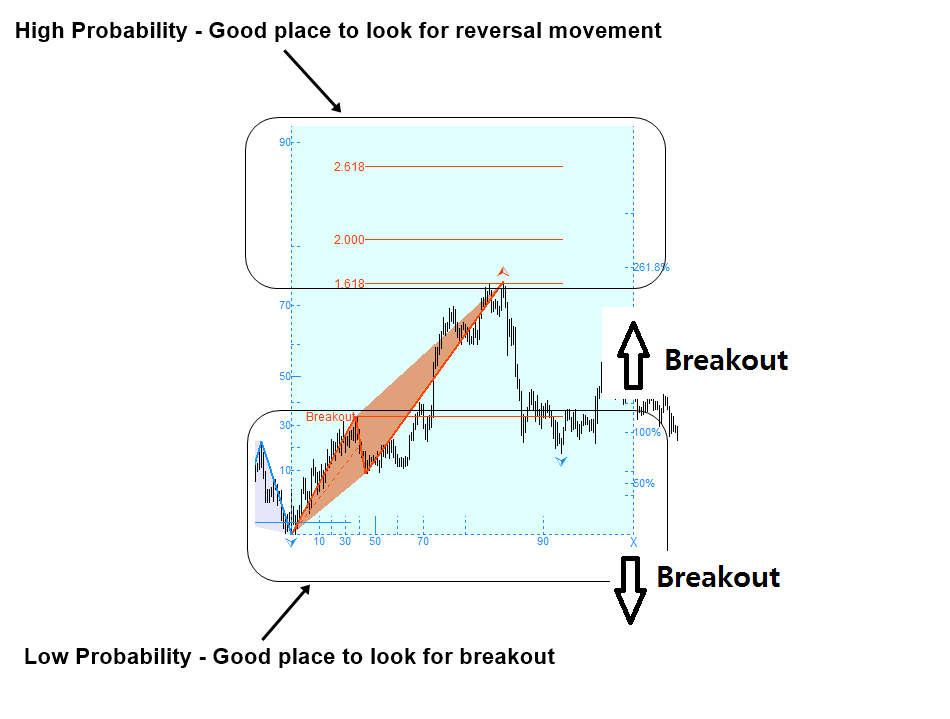# Predict Breakout using Technical Analysis

In trading, the turning point probability can be viewed as the first attempt to quantify the price action in the probabilistic manner. In science, it is the pioneering concept towards modelling the stochastic cycle. Practically speaking, the method helps with market timing for our trading in the quantitative way. In any sort of cycle, when the object rises sufficiently high, it should hit the peak and it should fall towards trough. The turning point probability applies the same principle in Forex and Stock market data. Hence, the turning point probability answers the question “How close the price is to peak so the price will start to fall towards trough?” This is an important question to ask for the trader because this prediction often helps us to make money.

We can not answer the question in the deterministic sense. However, we can answer the question in the probabilistic sense. Higher turning point probability means that the price is close to the peak. So we have a high chance to see that price makes a turn. For example, if the turning point probability is over 90%, there is a high chance that price can start to fall near the current price.

On the other hands, lower turning point probability means that the price is still far from the peak. Hence, there is a high chance for the price to continue to rise. For example, if the price is near 30% turning point probability, then we can say that there is a high chance for the price to go up. Of course, this is true in the cyclic sense only as the price will repeat up and down endlessly. However, this quantified information is still useful for our trading. In fact, for the convenience, one can use the turning point probability to get the trend probability. Simply, you can subtract the turning point probability from 100% to get the trend probability.

Trend Probability = 100% – Turning Point Probability (i.e. Rule of Thumb only)

Hence, as a rule of thumb, 30% turning point probability is equivalent to 70% (=100% -30%) trend probability as there is high chance for the price to continue to rise in this case as it is far from the peak.

So far, we have given the turning point examples for the case of peak or when price is moving to the peak. When we talk about measuring the turning point probability for trough, everything will be mirrored horizontally. In the case of trough, the turning point probability answers the question “How close the price is to trough so the price will start to rise towards peak?” If one can answer this question accurately, surely one can make good profits. To be useful, we can answer this question in the probabilistic manner.

Since the probability graph is mirrored for the case of trough, the turning point probability is measured from the peak to the latest price. Using this turning point probability, you can estimate if there is a high chance to make the turning point or to go down for the price in the cyclic sense. The good thing is that the turning point probability goes well with most of technical analysis. In the book “Science Of Support, Resistance, Fibonacci Analysis, Harmonic Pattern, Elliott Wave and X3 Chart Pattern: In Forex and Stock Market Trading”, we have shown how to combine the turning point probability with support and resistance. When you combine the turning point probability with support and resistance, the turning point probability helps you to confirm the direction. For example, when the turning point probability is high at support, you can expect the price to turn and to rise at the support.

Likewise, when the turning point probability is high at resistance, you can expect the price to turn and to fall at the resistance. You can use over 50% turning point probability to confirm the trading direction. However, typically, to predict the reversal movement, it is better to have the higher turning point probability. In this approach, the turning point probability complements the power of support and resistance to predict the trading direction. This is a good trading strategy to avoid or to reduce losing trades from the false breakout and false reversal when using the support and resistance. Likewise, in this book, we will show how to combine the turning point probability with supply and demand analysis to improve its performance. This would be the good demonstration toward using the probability for the practical trading.

This video explains the Breakout Pattern and Breakout Trading signals generated from analyzing Fractal Waves of the Financial Market. Analyzing Fractal Wave provides us the mean of predicting stochastic cycles in the financial market. Fractal Pattern Scanner analyzes the underlying Fractal Waves in the price series. Then, it will detect breakout patterns with high winning opportunity. These signals will provide the good entry and exit timing for high probability trading setup. In this video, we will talk about the signal panels of the breakout pattern across multiple timeframe.